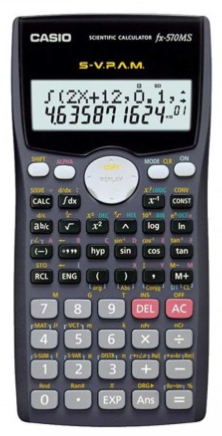Casio FX-570MS Price in BD | Casio Calculator | RYANS

# Casio FX-570MS Scientific Calculator #C19*Image may differ with actual product's layout, color, size & dimension. No claim will be accepted for image mismatch.

# Casio FX-570MS Scientific Calculator #C19

Product Id: 130.01.685.45

Regular Price  1,250
Special Price  1,200

Availability?

Quick Overview

Model - Casio FX-570MS
Brand - Casio
Type - Scientific Calculator
Display - Dot matrix display
Digit - 12 digits (10 + 2 digits)
Key - Plastic keys
Model
Casio FX-570MS
Type
Scientific Calculator
Display
Dot Matrix Display
Memory
No
Digit
12 (10 + 2 Digits)
Key
Plastic keys
Number of Functions
401
TAX Calculation
No
Power Sources
1 x LR44 Battery
Dimensions
155 x 78 x 13mm
Weight (Kg)
105gm
Specialty
Fraction calculations, Combination and permutation, Statistics (STAT-data editor, Standard deviation, Regression analysis), 9 variable memories, Comes with slide-on hard case, 10 + 2 digits, Dot matrix display, Regular percent, Multi-replay, Plastic keys, S-V.P.A.M. (Super Visually Perfect Algebraic Method), STAT-data editor, Scientific Constants, Vector, Base-n calculation, Button-type Battery, Basic Mathematical Functions, Basic Statistics, Metric conversion function, Non Graphing, Matrix, Equation, Integration, Complex number calculation, Differentiation
Others
Part No: C19, 10 + 2 digits: 10-digit mantissa + 2-digit exponential display, Dot matrix display: High-resolution screen provides beautiful looking graphs every time, Regular percent: Regular percentage calculations, Multi-replay: Quick and easy recall of previously executed formulas for editing and re-execution, Plastic keys: Designed and engineered for easy operation, S-V.P.A.M. (Super Visually Perfect Algebraic Method): All the features of the existing V.P.A.M. series plus a new 2-line display and a useful Replay function, STAT-data editor: Back-step viewing and editing of input data, Scientific Constants: can use constant symbols used in physics, science classes, Base-n calculation: Binary/Octal/Hexadecimal, Basic Mathematical Functions: Trigonometric, Exponential logarithmic, etc., Basic Statistics: Standard statistics functions such as Mean, SUM, Standard Deviation, and Regression, Metric conversion function: can convert a number in one unit to a number in a different unit
Warranty
3 year
Thailand
Country Of Origin
Japan

## fx-570MS## Features

• Fraction calculations
• Combination and permutation
• Statistics (STAT-data editor, Standard deviation, Regression analysis)
• 9 variable memories
• Comes with slide-on hard case

### fx-82MS/85MS/350MS functions, in addition to:

• Equation calculations
• Integration/differential calculations
• Base-n calculations/conversions
• Complex number calculations
• Matrix calculations
• Vector calculations
• 40 scientific constants

Brand - Casio, Model - Casio FX-570MS, Type - Scientific Calculator, Digit - 12 (10 + 2 Digits), Display - Dot Matrix Display, Key - Plastic keys, Number of Functions - 401, TAX Calculation - No, Memory - No, Power source - 1 x LR44 Battery, Dimension - 155 x 78 x 13mm, Weight - 105gm, Others - Part No: C19, 10 + 2 digits: 10-digit mantissa + 2-digit exponential display, Dot matrix display: High-resolution screen provides beautiful looking graphs every time, Regular percent: Regular percentage calculations, Multi-replay: Quick and easy recall of previously executed formulas for editing and re-execution, Plastic keys: Designed and engineered for easy operation, S-V.P.A.M. (Super Visually Perfect Algebraic Method): All the features of the existing V.P.A.M. series plus a new 2-line display and a useful Replay function, STAT-data editor: Back-step viewing and editing of input data, Scientific Constants: can use constant symbols used in physics, science classes, Base-n calculation: Binary/Octal/Hexadecimal, Basic Mathematical Functions: Trigonometric, Exponential logarithmic, etc., Basic Statistics: Standard statistics functions such as Mean, SUM, Standard Deviation, and Regression, Metric conversion function: can convert a number in one unit to a number in a different unit, Specialty - Fraction calculations, Combination and permutation, Statistics (STAT-data editor, Standard deviation, Regression analysis), 9 variable memories, Comes with slide-on hard case, 10 + 2 digits, Dot matrix display, Regular percent, Multi-replay, Plastic keys, S-V.P.A.M. (Super Visually Perfect Algebraic Method), STAT-data editor, Scientific Constants, Vector, Base-n calculation, Button-type Battery, Basic Mathematical Functions, Basic Statistics, Metric conversion function, Non Graphing, Matrix, Equation, Integration, Complex number calculation, Differentiation, Warranty - 3 year, Country of Origin - Japan, Made in/ Assemble - Thailand